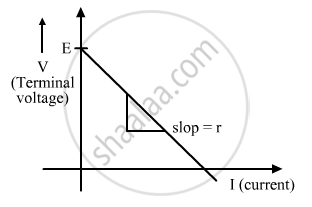Share

A Cell of Emf 'E' and Internal Resistance 'R' is Connected Across a Variable Resistor 'R'. Plot a Graph Showing Variation of Terminal Voltage 'V' of the Cell Versus the Current 'I'. - CBSE (Science) Class 12 - Physics

ConceptCells, Emf, Internal Resistance

Question

A cell of emf 'E' and internal resistance 'r' is connected across a variable resistor 'R'. Plot a graph showing variation of terminal voltage 'V' of the cell versus the current 'I'. Using the plot, show how the emf of the cell and its internal resistance can be determined.

Solution

Terminal voltage 'V' of the cell is  V = E − Ir

E is the emf of the cell, r is the internal resistance of the cell and I is the current through the circuit.

So, V = −Ir+E

Comparing with the equation of a straight line y = mx+c, we get:

y = V; x = I; m = −r; c = E

Graph showing variation of terminal voltage 'V' of the cell versus the current 'I' isEmf of the cell = Intercept on V axis
Internal resistance = slop of line.

Is there an error in this question or solution?

Video TutorialsVIEW ALL 

Solution A Cell of Emf 'E' and Internal Resistance 'R' is Connected Across a Variable Resistor 'R'. Plot a Graph Showing Variation of Terminal Voltage 'V' of the Cell Versus the Current 'I'. Concept: Cells, Emf, Internal Resistance.
S